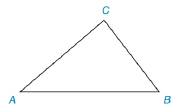Chapter 1.6, Problem 9E### Elementary Geometry for College St...

6th Edition
Daniel C. Alexander + 1 other
ISBN: 9781285195698

#### Solutions

Chapter
Section### Elementary Geometry for College St...

6th Edition
Daniel C. Alexander + 1 other
ISBN: 9781285195698
Textbook Problem
1 views

# In Exercise 5 to 9, use a compass and a straightedge to complete the constructions. Given: Triangle A B C Construction: The perpendicular bisector of sides A B → , A C → and B C →To determine

To find:

The complete construction of the perpendicular bisector of sides AB, AC and BC.

Explanation

Given:

A triangle ABC is given.

Construction:

A triangle ABC is given.

The steps are given below for the complete construction.

a) Use A, B and C as the centers for the compass and take the equal radius such that these circles should intersect with six points.

b) Mark the six points as D, E, F, H, I, F and G

### Still sussing out bartleby?

Check out a sample textbook solution.

See a sample solution

#### The Solution to Your Study Problems

Bartleby provides explanations to thousands of textbook problems written by our experts, many with advanced degrees!

Get Started

#### Find more solutions based on key concepts